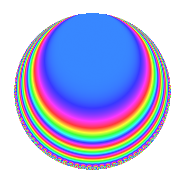# Properties

 Label 4032.2.j.bLevel 4032 Weight 2 Character orbit 4032.j Analytic conductor 32.196 Analytic rank 0 Dimension 4 CM No Inner twists 4

# Related objects

## Newspace parameters

 Level: $$N$$ = $$4032 = 2^{6} \cdot 3^{2} \cdot 7$$ Weight: $$k$$ = $$2$$ Character orbit: $$[\chi]$$ = 4032.j (of order $$2$$ and degree $$1$$)

## Newform invariants

 Self dual: No Analytic conductor: $$32.195682095$$ Analytic rank: $$0$$ Dimension: $$4$$ Coefficient field: $$\Q(\zeta_{8})$$ Coefficient ring: $$\Z[a_1, \ldots, a_{11}]$$ Coefficient ring index: $$2\cdot 3^{2}$$ Sato-Tate group: $\mathrm{SU}(2)[C_{2}]$

## $q$-expansion

Coefficients of the $$q$$-expansion are expressed in terms of a basis $$1,\beta_1,\beta_2,\beta_3$$ for the coefficient ring described below. We also show the integral $$q$$-expansion of the trace form.

 $$f(q)$$ $$=$$ $$q -\beta_{1} q^{7} +O(q^{10})$$ $$q -\beta_{1} q^{7} -\beta_{2} q^{11} -6 \beta_{1} q^{13} -\beta_{3} q^{23} -5 q^{25} -\beta_{3} q^{29} -4 \beta_{1} q^{31} + 6 \beta_{1} q^{37} + 2 \beta_{2} q^{41} -6 q^{43} -2 \beta_{3} q^{47} - q^{49} + 3 \beta_{3} q^{53} + 2 \beta_{2} q^{59} + 6 \beta_{1} q^{61} + 12 q^{67} -\beta_{3} q^{71} -2 q^{73} -\beta_{3} q^{77} -10 \beta_{1} q^{79} + 4 \beta_{2} q^{83} + 2 \beta_{2} q^{89} -6 q^{91} -10 q^{97} +O(q^{100})$$ $$\operatorname{Tr}(f)(q)$$ $$=$$ $$4q + O(q^{10})$$ $$4q - 20q^{25} - 24q^{43} - 4q^{49} + 48q^{67} - 8q^{73} - 24q^{91} - 40q^{97} + O(q^{100})$$

Basis of coefficient ring:

 $$\beta_{0}$$ $$=$$ $$1$$ $$\beta_{1}$$ $$=$$ $$\zeta_{8}^{2}$$ $$\beta_{2}$$ $$=$$ $$3 \zeta_{8}^{3} + 3 \zeta_{8}$$ $$\beta_{3}$$ $$=$$ $$-3 \zeta_{8}^{3} + 3 \zeta_{8}$$
 $$1$$ $$=$$ $$\beta_0$$ $$\zeta_{8}$$ $$=$$ $$($$$$\beta_{3} + \beta_{2}$$$$)/6$$ $$\zeta_{8}^{2}$$ $$=$$ $$\beta_{1}$$ $$\zeta_{8}^{3}$$ $$=$$ $$($$$$-\beta_{3} + \beta_{2}$$$$)/6$$

## Character Values

We give the values of $$\chi$$ on generators for $$\left(\mathbb{Z}/4032\mathbb{Z}\right)^\times$$.

 $$n$$ $$127$$ $$577$$ $$1793$$ $$3781$$ $$\chi(n)$$ $$-1$$ $$1$$ $$-1$$ $$-1$$

## Embeddings

For each embedding $$\iota_m$$ of the coefficient field, the values $$\iota_m(a_n)$$ are shown below.

For more information on an embedded modular form you can click on its label.

Label $$\iota_m(\nu)$$ $$a_{2}$$ $$a_{3}$$ $$a_{4}$$ $$a_{5}$$ $$a_{6}$$ $$a_{7}$$ $$a_{8}$$ $$a_{9}$$ $$a_{10}$$
2591.1
 0.707107 + 0.707107i −0.707107 − 0.707107i −0.707107 + 0.707107i 0.707107 − 0.707107i
0 0 0 0 0 1.00000i 0 0 0
2591.2 0 0 0 0 0 1.00000i 0 0 0
2591.3 0 0 0 0 0 1.00000i 0 0 0
2591.4 0 0 0 0 0 1.00000i 0 0 0
 $$n$$: e.g. 2-40 or 990-1000 Significant digits: Format: Complex embeddings Normalized embeddings Satake parameters Satake angles

## Inner twists

Char. orbit Parity Mult. Self Twist Proved
1.a Even 1 trivial yes
3.b Odd 1 yes
8.d Odd 1 yes
24.f Even 1 yes

## Hecke kernels

This newform can be constructed as the intersection of the kernels of the following linear operators acting on $$S_{2}^{\mathrm{new}}(4032, [\chi])$$:

 $$T_{5}$$ $$T_{19}$$ $$T_{43} + 6$$